PDE Solver VI

LabVIEW 2018 Help

Edition Date: March 2018
Part Number: 371361R-01
View Product InfoLabVIEW 2016 HelpLabVIEW 2017 HelpLabVIEW 2018 HelpLabVIEW 2019 HelpLabVIEW 2020 Help

Owning Palette: Partial Differential Equations VIs

Requires: Full Development System

Solves a partial differential equation. You must manually select the polymorphic instance to use.

Use the pull-down menu to select an instance of this VI.

 Select an instance 2D Helmholtz (Rectangular domain)2D Helmholtz (Polygonal domain)1D Heat2D Heat (Rectangular domain)1D Wave2D Wave (Rectangular domain)

2D Helmholtz (Rectangular domain)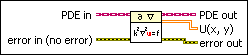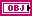PDE in is the class that stores the data of the equation.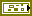error in describes error conditions that occur before this node runs. This input provides standard error in functionality.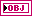PDE out returns PDE in unchanged.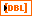U(x, y) returns the calculated solution of the equation. The size of the right-sided function is # of y-points-by-# of x-points from the Define PDE Domain VI. Each column and row of U(x, y) stores the solution on a particular y- or x-point, respectively.error out contains error information. This output provides standard error out functionality.

2D Helmholtz (Polygonal domain)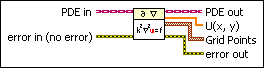PDE in is the class that stores the data of the equation.error in describes error conditions that occur before this node runs. This input provides standard error in functionality.PDE out returns PDE in unchanged.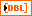U(x, y) returns the calculated solution of the equation at Grid Points.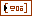Grid Points returns the grid points for the equation.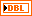X returns the x-coordinate of Grid Points.Y returns the y-coordinate of Grid Points.error out contains error information. This output provides standard error out functionality.

1D Heat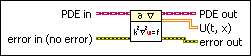PDE in is the class that stores the data of the equation.error in describes error conditions that occur before this node runs. This input provides standard error in functionality.PDE out returns PDE in unchanged.U(t, x) returns the calculated solution of the equation. The size of the right-sided function is # of t-points-by-# of x-points from the Define PDE Domain VI. Each row of U(t, x) stores the solution at points X from the Define PDE Domain VI at a particular time step. Each column of U(t, x) stores the solution on a particular x-point over time.error out contains error information. This output provides standard error out functionality.

2D Heat (Rectangular domain)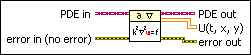PDE in is the class that stores the data of the equation.error in describes error conditions that occur before this node runs. This input provides standard error in functionality.PDE out returns PDE in unchanged.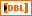U(t, x, y) returns the calculated solution of the equation. The size of the right-sided function is # of t-points-by-# of y-points-by-# of x-points from the Define PDE Domain VI. Each page of U(t, x, y) stores the solution at mesh grid points (X, Y) from the Define PDE Domain VI at a particular time step. On one page of U(t, x, y), each row or column stores the solution on a particular y- or x-point, respectively.error out contains error information. This output provides standard error out functionality.

1D WavePDE in is the class that stores the data of the equation.error in describes error conditions that occur before this node runs. This input provides standard error in functionality.PDE out returns PDE in unchanged.U(t, x) returns the calculated solution of the equation. The size of the right-sided function is # of t-points-by-# of x-points from the Define PDE Domain VI. Each row of U(t, x) stores the solution at points X from the Define PDE Domain VI at a particular time step. Each column of U(t, x) stores the solution on a particular x-point over time.error out contains error information. This output provides standard error out functionality.

2D Wave (Rectangular domain)PDE in is the class that stores the data of the equation.error in describes error conditions that occur before this node runs. This input provides standard error in functionality.PDE out returns PDE in unchanged.U(t, x, y) returns the calculated solution of the equation. The size of the right-sided function is # of t-points-by-# of y-points-by-# of x-points from the Define PDE Domain VI. Each page of U(t, x, y) stores the solution at mesh grid points (X, Y) from the Define PDE Domain VI at a particular time step. On one page of U(t, x, y), each row or column stores the solution on a particular y- or x-point, respectively.error out contains error information. This output provides standard error out functionality.

PDE Solver Details

Before you use the PDE Solver VI, use the Define PDE, Define PDE Domain, and Define PDE Boundary Condition VIs to define the equation, domain, and boundary conditions. For an evolutionary equation, such as the heat or wave equations, use the Define PDE Initial Condition VI to define the initial condition. For each VI, select the appropriate polymorphic instance according to the type of equation you need to solve. The following block diagram illustrates how to define and solve a one-dimensional wave equation.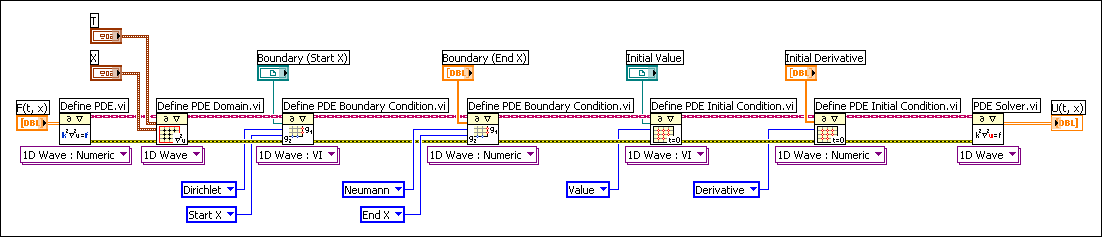For a one-dimensional problem, LabVIEW solves the equation on evenly spaced points with the finite difference method. For a two-dimensional problem defined on the rectangular domain, LabVIEW solves the equation on a uniform mesh grid with the finite difference method. For a two-dimensional problem defined on the polygonal domain, LabVIEW solves the equation on specified grid points with the finite element method. In this case, the grid points are not required to be a uniform mesh.

Examples

Refer to the following VIs for examples of using the PDE Solver VI:

• PDE Flexible Element VI: labview\examples\Mathematics\Differential Equations - PDE
• PDE String Vibration VI: labview\examples\Mathematics\Differential Equations - PDE
• PDE Thermal Distribution VI: labview\examples\Mathematics\Differential Equations - PDE